Anzeige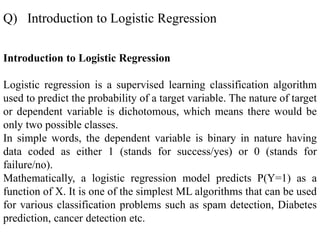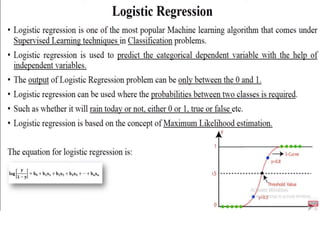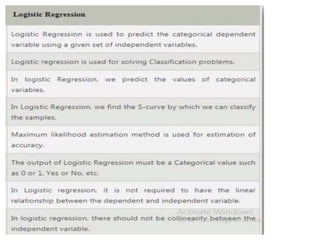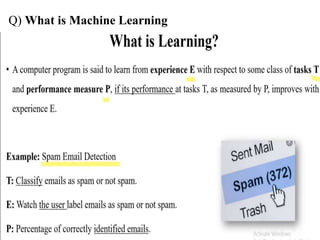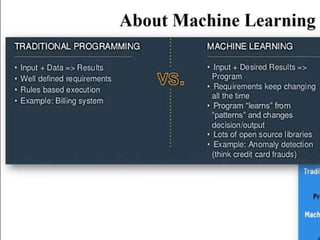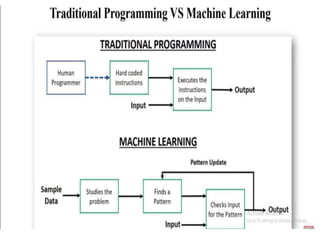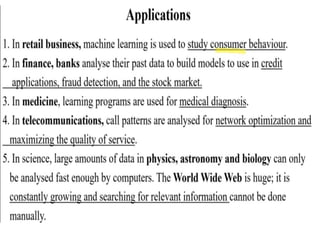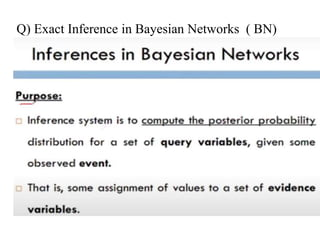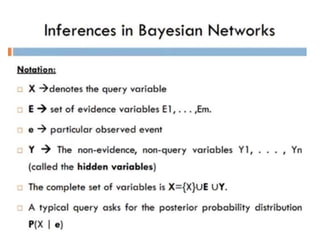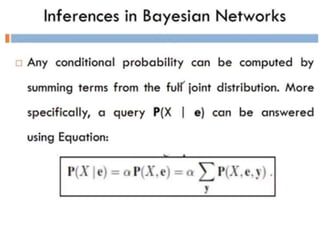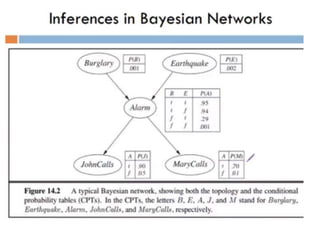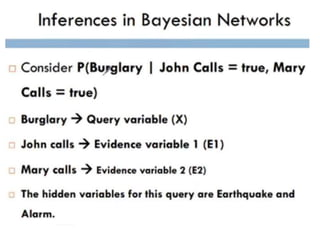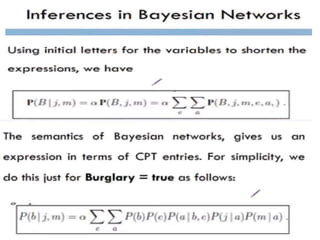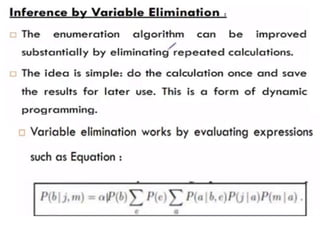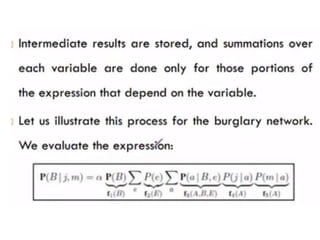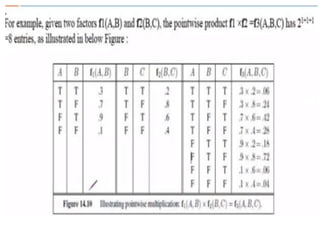1 von 19
Anzeige

### Easy notes .ppt

1. Introduction to Logistic Regression Logistic regression is a supervised learning classification algorithm used to predict the probability of a target variable. The nature of target or dependent variable is dichotomous, which means there would be only two possible classes. In simple words, the dependent variable is binary in nature having data coded as either 1 (stands for success/yes) or 0 (stands for failure/no). Mathematically, a logistic regression model predicts P(Y=1) as a function of X. It is one of the simplest ML algorithms that can be used for various classification problems such as spam detection, Diabetes prediction, cancer detection etc. Q) Introduction to Logistic Regression
2. Q) What is Machine Learning
3. Q) Exact Inference in Bayesian Networks ( BN)
Anzeige